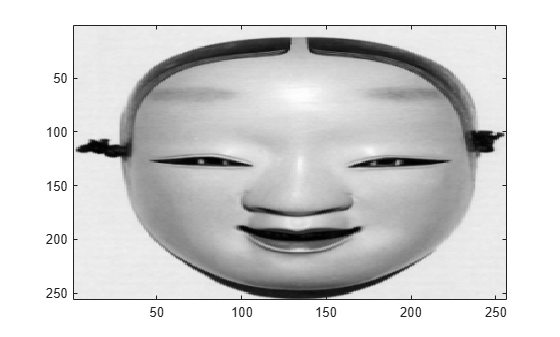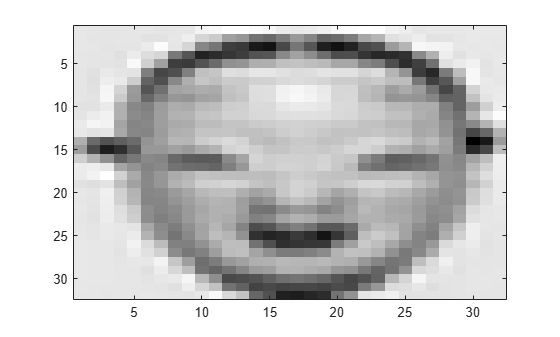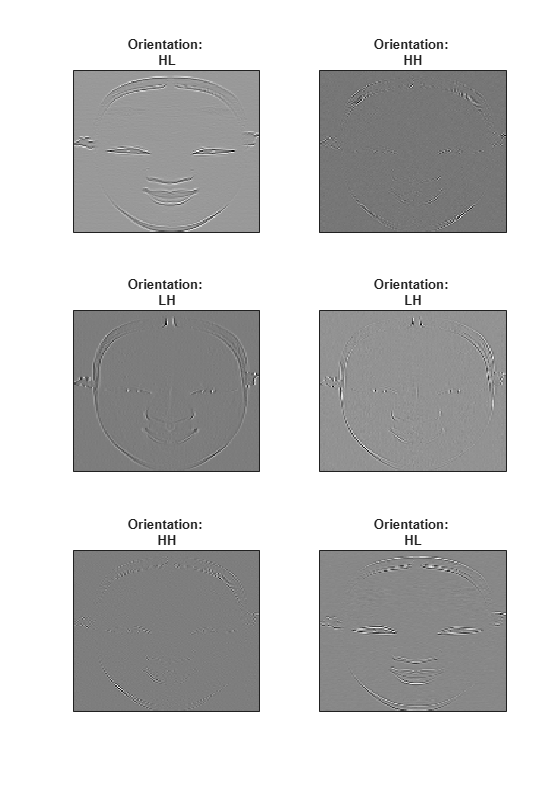# dualtree2

Kingsbury Q-shift 2-D dual-tree complex wavelet transform

Since R2020a

## Syntax

``[A,D] = dualtree2(X)``
``[___,Ascale] = dualtree2(X)``
``[___] = dualtree2(X,Name,Value)``

## Description

````[A,D] = dualtree2(X)` returns the 2-D dual-tree complex wavelet transform (DTCWT) of `X` using Kingsbury Q-shift filters. The output `A` is the matrix of real-valued final-level scaling (lowpass) coefficients. The output `D` is a L-by-1 cell array of complex-valued wavelet coefficients, where L is the level of the transform. For each element of `D` there are six wavelet subbands.The DTCWT is obtained by default down to level ```floor(log2(min([H W])))```, where H and W refer to the height (row dimension) and width (column dimension) of `X`, respectively. If any of the row or column dimensions of `X` are odd, `X` is extended along that dimension by reflecting around the last row or column.By default, `dualtree2` uses the near-symmetric biorthogonal wavelet filter pair with lengths 5 (scaling filter) and 7 (wavelet filter) for level 1 and the orthogonal Q-shift Hilbert wavelet filter pair of length 10 for levels greater than or equal to 2.```
````[___,Ascale] = dualtree2(X)` returns the scaling (lowpass) coefficients at each level.```

example

````[___] = dualtree2(X,Name,Value)` specifies additional options using name-value pair arguments. For example, `'LevelOneFilter','antonini'` specifies the (9,7)-tap Antonini filter as the biorthogonal filter to use in the first-level analysis.```

## Examples

collapse all

```load mask imagesc(X) colormap gray```Obtain the dual-tree complex wavelet transform of the image down to four levels of resolution.

`[a,d] = dualtree2(X,'Level',4);`

Display the final-level scaling (lowpass) coefficients.

```imagesc(a) colormap gray```Display the tree B wavelet coefficients at the finest scale. Each subplot title denotes the particular subband ("H" for highpass, "L" for lowpass).

```orientation = ["HL","HH","LH","LH","HH","HL"]; for k=1:6 subplot(3,2,k) imagesc(imag(d{1}(:,:,k))) title(['Orientation: ' orientation(k)]) set(gca,'xtick',[]) set(gca,'ytick',[]) end colormap gray set(gcf,'Position',[0 0 560 800])```## Input Arguments

collapse all

Input data, specified as a real-valued matrix, 3-D array, or 4-D array. `X` is a real-valued H-by-W-by-C-by-N array, where H is the height or row dimension, W is the width or column dimension, C is the number of channels, and N is the number of images. `X` must have at least two samples in each of the row and column dimensions.

Example: If `X` is a 256-by-256-by-3-by-2 array, `X` contains two 256-by-256 RGB images.

Data Types: `double` | `single`

### Name-Value Arguments

Specify optional pairs of arguments as `Name1=Value1,...,NameN=ValueN`, where `Name` is the argument name and `Value` is the corresponding value. Name-value arguments must appear after other arguments, but the order of the pairs does not matter.

Before R2021a, use commas to separate each name and value, and enclose `Name` in quotes.

Example: `'LevelOneFilter','antonini','Level',4`

Level of decomposition, specified as a positive integer less than or equal to ```floor(log2(min([H W])))```, where H and W refer to the height (row dimension) and width (column dimension) of `X`, respectively. If unspecified, `Level` defaults to ```floor(log2(min([H W])))```.

Biorthogonal filter to use in the first-level analysis, specified as:

• `'legall'` — LeGall 5/3 filter 

• `'nearsym13_19'` — (13,19)-tap near-orthogonal filter 

• `'nearsym5_7'` — (5,7)-tap near-orthogonal filter 

• `'antonini'` — (9,7)-tap Antonini filter 

By default, `dualtree2` uses `'nearsym5_7'`, the near-symmetric biorthogonal filter pair with lengths 5 (scaling filter) and 7 (wavelet filter).

Orthogonal Hilbert Q-shift analysis filter pair length to use for levels 2 and higher, specified as one of the listed values . By default, `dualtree2` uses the orthogonal Q-shift Hilbert wavelet filter pair of length 10.

## Output Arguments

collapse all

Final-level approximation coefficients, returned as a real-valued matrix.

Wavelet coefficients, returned as an L-by-1 cell array of complex-valued wavelet coefficients, where L is the level of the transform. The real parts of the coefficients are from tree A, and the imaginary parts are from tree B. For each element of `D` there are six wavelet subbands.

Approximation coefficients at each level of the transform, returned as an L-by-1 cell array of real-valued scaling (lowpass) coefficients, where L is the level of the transform. If `X` is a matrix, each element of `D` is a matrix whose column dimension equals the column dimension of `X`.

 Antonini, M., M. Barlaud, P. Mathieu, and I. Daubechies. “Image Coding Using Wavelet Transform.” IEEE Transactions on Image Processing 1, no. 2 (April 1992): 205–20. https://doi.org/10.1109/83.136597.

 Kingsbury, Nick. “Complex Wavelets for Shift Invariant Analysis and Filtering of Signals.” Applied and Computational Harmonic Analysis 10, no. 3 (May 2001): 234–53. https://doi.org/10.1006/acha.2000.0343.

 Le Gall, D., and A. Tabatabai. “Sub-Band Coding of Digital Images Using Symmetric Short Kernel Filters and Arithmetic Coding Techniques.” In ICASSP-88., International Conference on Acoustics, Speech, and Signal Processing, 761–64. New York, NY, USA: IEEE, 1988. https://doi.org/10.1109/ICASSP.1988.196696.